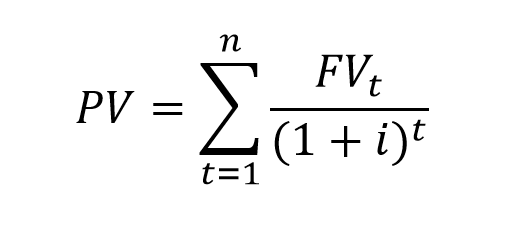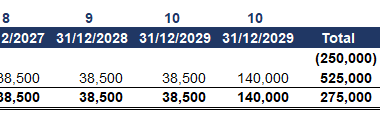## Introduction

To understand the Time Value of Money, imagine you were offered 100 euros now or 100 euros in three years, what would you prefer? If you are like me, you’d probably prefer the money now. But why is that, when a 100 euros has the same value now and in the future?

Having it now makes it more valuable, as we can use it to generate returns in the following three years.

If we invest a 100 euro today, at a 3% annual interest and reinvest for the next three years, in the end, we will have about 109 euro. The difference to 100 euro is known as the Time Value of Money.

And what if we are offered 200 euros now or 220 euros in three years? How do we decide? We can calculate the future value of 200 euros and compare it to 220 euros in three years.

Using the same interest rate of 3% we get roughly 219 euros at the end of the period. This means that the alternative of receiving 220 euros in three years appears to be a better option.

Let’s explore the concept of Time Value of Money and see how to calculate present and future values of cash flows.

## The Time Value of Money (TMV) ConceptYou are probably familiar with the saying that time is money. The Time Value of Money is a paramount financial concept. A certain amount now is worth more than the same amount in the future. This is because we can invest now and earn a return, resulting in more money in the future. Another reason is that a promise for future cash flows always carries the risk of default.

We can also refer to the concept as the Net Present Value (NPV) of money.

The same amount now and in the future has a different value. And it is important to know how to calculate the time value of money so that we can better compare investments with varying periods.

If someone offers us 1,000 euros now or 1,100 euros in one year, the critical question is, can we make a 10% return in one year. Can we invest the 1,000 euro in such a way that it will generate a 100 euro return in one year?

We should always look at The Time Value of Money considering inflation and the resulting adjustment to purchasing power.

Inflation harms our ability to buy goods. By decreasing the value of money, inflation diminishes the purchasing power we have, given no change in the funds available.

Therefore we need to consider inflation when calculating the real Return on Investment (ROI) of a project. If the computed profitability is less than inflation, we can end up in a situation where we are making money, but losing purchasing power.

## Time Value of Money Formulas

There are two ways we can calculate the Time Value of Money. We can find the present value (PV) of future cash flow via the following formula:Where:

• PV – Present Value;
• FV – Future Value;
• i – interest rate;
• n – number of periods.

Mathematically, we can express this in a more visually compact form:Notice the negative sign of the power, which allows us to remove the fractions from the equation.

The following formula allows us to calculate the future value (FV) of cash flow from its present value.Where:

• FV – Future Value;
• PV – Present Value;
• i – interest rate;
• n – number of periods.

In cases where we have more than one compounding period of interest per year, we can tweak the formula, to make sure we are using the appropriate portion of annual interest:Where:

• FV – Future Value;
• PV – Present Value;
• i – interest rate (annual);
• t – number of periods (years);
• n – number of compounding periods of interest per year.

Time Value of Money is a fundamental underlying concept for calculating Net Present Value (NPV), Compound Annual Growth Rate (CAGR), Internal Rate of Return (IRR), and others.

When performing an NPV calculation, we can use the formula to calculate the present value of future cash flows:Where:

• PV – Present Value;
• FV – Future Value;
• t – current period;
• n – number of periods;
• i – interest rate.

We can use the general formulas of the concept for any series of cash flows.

We can calculate the metrics around the Time Value of Money using financial calculators, or a spreadsheet application like Excel. You can read more on the business functions, or look in the Office docs for the following specific ones – PV, FV, IRR, NPV.

One of the most critical moments when performing Time Value of Money calculations is selecting the appropriate rate of return (discount rate). One of the popular choices for rate, apart from interest on the debt, is the Weighted Average Cost of Capital (WACC). It is essential to be aware that incorrect choice of a rate will probably make the whole exercise meaningless and can have a severely adverse impact on our decision-making process.

## Example

To illustrate the concept of Time Value of Money, we will look at the following example. We are looking to invest in a machine that will give us 38,500 euros in annual benefits for the next ten years. The device will have an initial investment cost of 250 thousand euros, and after the useful life has passed, we can sell the machine for 140 thousand euros.We can build a simple schedule to represent our cash flows per period. To keep the example compact, we will assume inflation is at 0% over the period. We start with the initial CAPEX and list the cash benefit per annum.At the end of our table, we add two columns, with the same end dates, to show the annual benefit and the release value separately at the end of the ten years.Looking at it like that, we can immediately see that we will get twice the initial investment amount over the years. But to gain a better picture, we need to consider the Time Value of Money.

We can use the company’s Weighted Average Cost of Capital (WACC) as a discount rate, as it represents best the actual cost of capital for the enterprise.

We can now employ the more sophisticated XNPV formula in Excel to calculate the Net Present Value of the cash flows.The computation gives us a positive NPV of 33 thousand euros. We can conclude that the project is economically viable, based on the company’s specific cost of capital.

## ConclusionThe Time Value of Money concept is crucial in calculating the intrinsic value of shares and investment opportunities in companies and projects. Almost every recommendation ever given is based on the TVM concept, even if the person making it isn’t aware.

Because of this concept, we know that the earlier we start to invest, the better. It is the benefit of receiving money now instead of in the future that makes investing work, and it is rooted in time preference. In the end, Time Value of Money explains well the reason behind the existence of interest rates.

If you liked the article, you could show your support by sharing it with colleagues and friends. Also, don’t forget to download the Excel model below.## FCCA, FMVA

Hi! I am a finance professional with 10+ years of experience in audit, controlling, reporting, financial analysis and modeling. I am excited to delve deep into specifics of various industries, where I can identify the best solutions for clients I work with.

In my spare time, I am into skiing, hiking and running. I am also active on Instagram and YouTube, where I try different ways to express my creative side.